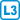## Alternative axiomatization II

Let $$(X, \mathcal P)$$ be a set system and for $$n \in \mathbb N$$, $$n \geq 2$$, holds that:

1. $$|X|=|\mathcal P|=n^2+n+1$$,
2. $$\forall P \in \mathcal P: |P|=n+1$$,
3. $$\forall P,Q\in\mathcal P: P \ne Q \Rightarrow |P \cap Q| \leq 1$$.

Is then $$(X, \mathcal P)$$ a finite projective plane of order $$n$$?

• #### Hint

Compare the number of all pairs of points with the number of pairs that define a line to prove that every two points uniquely identify a line.

• #### Solution

It follows from axiom (2) and the condition $$n \ge 2$$ that each line has at least three points. We choose the lines $$P,Q$$ arbitrarily, then due to (3) $$P$$ contains points $$a, b$$ not lying on $$Q$$ and the line $$Q$$ has points $$c, d$$ not lying on $$P$$. The four points $$C = \{a, b, c, d \}$$ are in the general position. If some line $$R$$ satisfies $$|R \cap C| \ge 3$$, then either $$| R \cap P | = 2$$ or $$|R \cap Q | = 2$$, which would be in conflict with the axiom (3).

On $$X$$ we can create $$\binom{|X|}{2} = \binom{n^2+n+1}{2}$$ pairs of points (1). Each line $$P$$ is determined by $$\binom{|P|}{2}=\binom{n+1}{2}$$ pairs of points (2).

Due to (3) one pair can determine at most one line. To get $$n^2+n+1$$ lines (1) we need at least $$(n^2+n+1)\binom{n+1}{2}$$ point pairs.

However, since $$(n^2+n+1)\binom{n+1}{2}=\binom{n^2+n+1}{2}$$, there is no pair of points left that would not determine a line. Thus, we proved the next axiom of the projective plane, namely that each pair of points uniquely determines a line.

Before proving the last axiom, we estimate the number of lines passing through any point $$a$$. In addition to $$a$$, on each line passing through $$a$$ there are $$n$$ points (2) from the remaining $$n^2+n$$ (1), and these points must be different (3). Thus, the point $$and$$ may lie on at most $$\frac{n^2+n}{n}=n+1$$ lines.

However, since $$n^2+n+1>n+1$$, there exists a line $$P: a\notin P$$ for the point $$a$$. Each point of $$P$$ determines, due to the just proved axiom, one line passing through $$a$$, i.e. $$a$$ belongs to at least $$n+1$$ lines.

(The existence of $$P$$ alternatively: choose two different lines $$Q, R$$ passing through $$a$$. If $$a$$ belongs only to one line, choose $$P$$ from the remaining $$n^2+n$$ lines arbitrarily. Else, on the lines $$Q, R$$ select the points $$b, c$$. These exist because $$n \ge 2$$ and (2). In the previous paragraph we have showed that the line $$P$$ determined by $$b, c$$ exists. It does not contan $$a$$ due to (3).)

Now let us similarly prove the axiom of the intersection of lines. According to (3) it suffices to show that every two lines intersect. There are $$\binom{n^2+n+1}{2}$$ pairs of lines. Each single point corresponds to the intersection of at least $$\binom{n+1}{2}$$ pairs of lines and since the total number of points is $$n^2+n+1$$, we find that no two lines remain that would not intersect.